M328K Final Exam, May 10, 2003

1. ``Bibonacci'' numbers. The Bibonacci numbers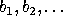are defined by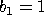,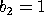, and, for n>2,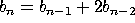.

a) Prove that, for all positive integers n,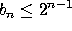.

b) Prove that, for all positive integers n,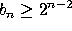.

2. The following theorem-proof combination is erroneous. Find the errors (there are at least two). Then, rewrite the proof (and, if necessary, the statement of the theorem) so that it really does prove the theorem.

``Theorem'' Every positive integer is divisible by a prime.

``Proof'' Let n be a positive integer. If n is prime, then n is divisible by the prime n, and we are done. If n is composite, then n = ab, where a and b are integers less than n. If a is prime, then a|n, and we are done. If a is composite, write a as a product of two smaller integers, and examine the first of these. Repeat this process, dividing composite factors into products of still smaller factors, and examining the first sub-factor, until you get a factor that cannot be divided further, and is therefore prime.

3. Matrix products. It is a known fact that, if A and B are matrices such that the product AB makes sense, then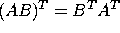. (That is, the transpose of the product is the product of the transposes, in the opposite order). Taking this fact as given, prove the following statement:

Claim: Let n > 1 and let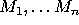be an ordered n-tuple of matrices such that the product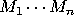is defined. Then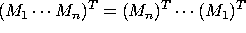, i.e., the transpose of the product is the product of the transposes with the order reversed.

4. a) Find the greatest common factor of 50 and 76.

b) Write this number as a linear combination of 50 and 76.

c) Find all solutions, mod 76, of the equation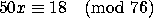.

5. a) Find all solutions, in the range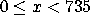, to the following system of congruences.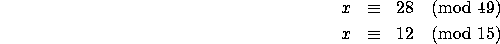b) Find all solutions, in the range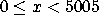, to the system of congruences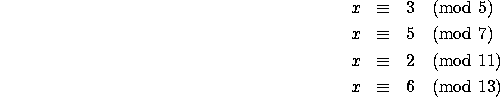6. a) Compute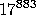(mod 101). (Note that 101 is prime)

b) You are told that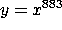(mod 101) and that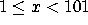. Find a positive integer d such that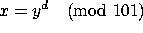.

c) If y=24, what is x?

7. Let n=8999271. a) What is the prime factorization of n? (Hint: n is the product of more than two primes) You may use your calculators, but you may NOT use a ``factor'' key or function, and you MUST show how you did the factorization in order to receive credit.

b) Compute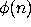.

c) Somebody suggests coding numbers by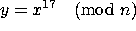, where x is relatively prime to n. Find a key that decodes this (i.e., a number k such that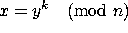.